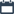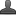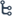•7:33 PM
•••No comments

## Bài 10. Hồi quy (Curve ﬁtting)

Matlab tìm ra hàm hồi quy của 1 đường cong bằng thuật toán ‘least squares curve ﬁtting’. The curve used is restricted to polynomials. With the command polyfit any polynomial can be ﬁtted to the data. polyfit (x, y, n)

ﬁnds the coeﬃcients of a polynomial of degree n that ﬁts the data (ﬁnds the linear relation between x and y).
Let’s start with ﬁnding a linear regression of some data:
>> x = 0:10;
>> y = [-.10 .24 1.02 1.58 2.84 2.76 2.99 4.05 4.83 5.22 7.51]
>> p = polyfit (x, y, 1) % find the fitting polynomial of order 1
p = 0.6772 -0.3914
The output of polyfit is a row vector of the polynomial coeﬃcients, the solution of this example is therefore
y = 0.6772x − 0.3914.
Exercise 51.

## Cảm nhận Học viên

Matlabthayhai. Powered by Blogger.